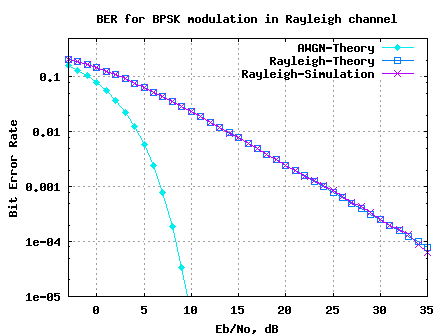- DSP log - http://www.dsplog.com -

BER for BPSK in Rayleigh channel

Posted By Krishna Sankar On August 10, 2008 @ 8:50 pm In Channel,Modulation | 282 Comments

Long back in time we discussed the BER (bit error rate) for BPSK modulation  in a simple AWGN channel (time stamps states August 2007). Almost an year back! It high time we discuss the BER for BPSK in a Rayleigh multipath channel.

In a brief discussion on Rayleigh channel , wherein we stated that a circularly symmetric complex Gaussian random variable is of the form,

,

where real and imaginary parts are zero mean independent and identically distributed (iid) Gaussian random variables with mean 0 and variance .

The magnitude which has a probability density,

is called a Rayleigh random variable. This model, called Rayleigh fading channel model, is reasonable for an environment where there are large number of reflectors.

## System model

, where
is complex scaling factor corresponding to Rayleigh multipath channel
is the transmitted symbol (taking values +1′s and -1′s) and
is the Additive White Gaussian Noise (AWGN)

Assumptions

1. The channel is flat fading – In simple terms, it means that the multipath channel has only one tap. So, the convolution operation reduces to a simple multiplication. For a more rigorous discussion on flat fading and frequency selective fading, may I urge you to review Chapter 15.3 Signal Time-Spreading from [DIGITAL COMMUNICATIONS: SKLAR]2. The channel is randomly varying in time – meaning each transmitted symbol gets multiplied by a randomly varying complex number . Since is modeling a Rayleigh channel, the real and imaginary parts are Gaussian distributed having mean 0 and variance 1/2.

3. The noise has the Gaussian probability density function with

with and .

4. The channel is known at the receiver. Equalization is performed at the receiver by dividing the received symbol by the apriori known i.e.

where
is the additive noise scaled by the channel coefficient.

## Bit Error Rate

The equations listed below refers Chapter 14.3 in [DIGITAL COMMUNICATION: PROAKIS]If you recall, in the post on BER computation in AWGN, the probability of error for transmission of either +1 or -1 is computed by integrating the tail of the Gaussian probability density function for a given value of bit energy to noise ratio . The bit error rate is,

.

However in the presence of channel , the effective bit energy to noise ratio is . So the bit error probability for a given value of is,

,

where .

To find the error probability over all random values of , one must evaluate the conditional probability density function over the probability density function of .

Probability density function of

From our discussion on chi-square random variable , we know that if is a Rayleigh distributed random variable, then is chi-square distributed with two degrees of freedom. since is chi square distributed, is also chi square distributed. The probability density function of is,

.

Error probability

So the error probability is,

.

Somehow, this equation reduces to

.

Note:

1. I have not yet figured out the math to reduce the above integral to the answer. If some one knows, kindly drop in a comment.
2. Another way for finding the bit error rate might be to find the pdf of . However, I do not know how to find pdf following the division of two random variables.## Simulation Model

It will be useful to provide a simple Matlab/Octave example simulating a BPSK transmission and reception in Rayleigh channel. The script performs the following

(a) Generate random binary sequence of +1′s and -1′s.

(b) Multiply the symbols with the channel and then add white Gaussian noise.

(c) At the receiver, equalize (divide) the received symbols with the known channel

(d) Perform hard decision decoding and count the bit errors

(e) Repeat for multiple values of and plot the simulation and theoretical results.Figure: BER plot of BPSK in Rayleigh fading channel

When compared to the AWGN case, around 25dB degradation due to the multipath channel (at the point). This is both good and bad: bad because we need to spend so much energy to get a reliable wireless link up (in this era of global warming), and good because we signal processing engineers are trying to figure out ways for improving the performance.

## Reference

[DIGITAL COMMUNICATION: PROAKIS]Digital Communications by John Proakis 

URL to article: http://www.dsplog.com/2008/08/10/ber-bpsk-rayleigh-channel/

URLs in this post:

 BER (bit error rate) for BPSK modulation: http://www.dsplog.com/2007/08/05/bit-error-probability-for-bpsk-modulation/

 discussion on Rayleigh channel: http://www.dsplog.com/2008/07/14/rayleigh-multipath-channel/